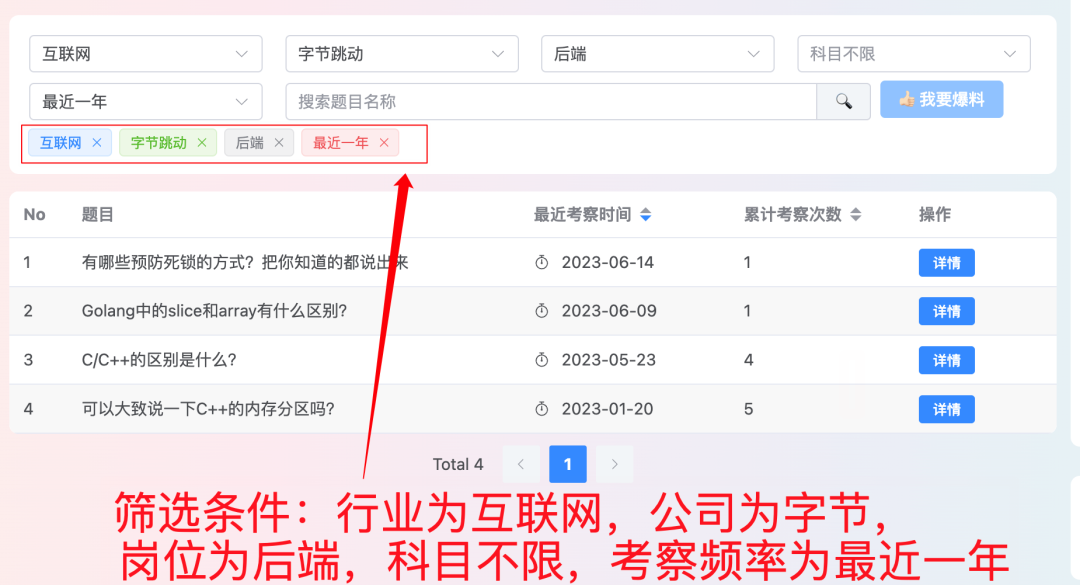0、经常看到有人问哪里有汇总好的校招投递公司信息汇总？甚至还有的人花钱去买这类信息，更有甚者还有被骗的。。。其实这类信息真的很多，牛客网、前程无忧这些招聘网站都帮你总结好了，这里分享一下一位学弟告知的前程无忧推出的校招信息汇总，他们每天都会在群里发招聘公司、行业、岗位等，点此免费获取前程无忧总结好的校招资讯汇总

1、👉23年5月份我从字节跳动离职跳槽到某外企期间，为方便自己找工作，增加上岸几率，我自己从0开发了一个大厂面试真题解析网站，包括两个前端和一个后端。能够定向查看某些公司的某些岗位面试真题，比如我想查一下行业为互联网，公司为字节跳动，考察岗位为后端，考察时间为最近一年之类的面试题有哪些？2、😍 免费分享阿秀个人学习计算机以来收集到的免费学习资源，点此白嫖；也记录一下自己以前买过的不错的计算机书籍、网络专栏和垃圾付费专栏

3、🚀如果你想在校招中顺利拿到更好的offer，阿秀建议你多看看前人踩过的坑留下的经验，事实上你现在遇到的大多数问题你的学长学姐师兄师姐基本都已经遇到过了。

4、🔥 欢迎准备计算机校招的小伙伴加入我的学习圈子，一个人踽踽独行不如一群人报团取暖，圈子里沉淀了很多过去21/22/23届学长学姐的经验和总结，好好跟着走下去的，最后基本都可以拿到不错的offer！此外，每周都会进行精华总结和分享！如果你需要《阿秀的学习笔记》网站中📚︎校招八股文相关知识点的PDF版本的话，可以点此下载

## # 636. 函数的独占时间

### # 第一版，看了别人的思路，真的厉害

`````` vector<int> exclusiveTime(int n, vector<string>& logs) {
vector<int> result(n, 0);
stack<pair<int, int>> myStack;//辅助栈，<first, second>分别代表<函数id，起始时间>
//扫描所有log
for (auto& log : logs) {
string operation = "";//id的操作，“start”或者“end”
int funcId = 0, timeStamp = 0, index = 0, logSize = log.size();//log的函数id、操作时间戳
//第一步：读取函数id
while (log[index] != ':') {
funcId = funcId * 10 + log[index++] - '0';
}
index += 1;//跳过 :
//第二步：读取操作类型
while (log[index] != ':') {
operation += log[index++];
}
index += 1; //再次跳过 :
//第三步：读取时间戳
while (index < logSize) {
timeStamp = timeStamp * 10 + log[index++] - '0';
}
//第四步：根据操作进行不同的处理
if (operation == "start") {
//如果是函数start，直接放入stack中
myStack.push({ funcId, timeStamp });
}
else {//函数end的情况
int runTime = timeStamp - myStack.top().second + 1;//计算栈顶id运行时间
myStack.pop();
result[funcId] += runTime;//加入到总时间中
//如果此时栈还不为空，则说明当前栈顶函数里面嵌套了当前函数id，需要减去当前函数运行的时间
if (!myStack.empty()) {
result[myStack.top().first] -= runTime;
}
}
}
return result;
}
``````
1
2
3
4
5
6
7
8
9
10
11
12
13
14
15
16
17
18
19
20
21
22
23
24
25
26
27
28
29
30
31
32
33
34
35
36
37
38

### # 第二版，自己复现一遍

``````vector<int> exclusiveTime(int n, vector<string>& logs) {//自己复现一编
vector<int> res(n,0);
stack<pair<int, int>>st;//函数ID和起始时间的映射

for (auto& log : logs) {

int funID = 0, startTime = 0, logSize=log.size(), index=0;
string opera= "";
while (log[index] != ':') {//取的函数ID
funID = funID * 10 + log[index++] - '0';
}
index++;//跳过 : 号
//cout << "funID" << funID << endl;
while (log[index] != ':') {//取的开始还是结束符号
opera +=  log[index++];
}
//cout << "opera" << opera << endl;
index++;//再次跳过 :号

while (index<logSize) {//取的操作时间
startTime = startTime * 10 + log[index++] - '0';
}
//cout << "startTime" << startTime << endl;
if (opera == "start") {//存入开始时间
st.push({ funID,startTime });
}
else {
int totalRunTime = startTime - st.top().second + 1;
//cout << "totaltime " << totalRunTime << endl;
res[funID] += totalRunTime;//以0为中介，属于自己的就加上则减
st.pop();
if (!st.empty()) {
res[st.top().first] -= totalRunTime;//不属于自己的就减去
}
}

}
return res;

}
``````
1
2
3
4
5
6
7
8
9
10
11
12
13
14
15
16
17
18
19
20
21
22
23
24
25
26
27
28
29
30
31
32
33
34
35
36
37
38
39
40

### # 第三种，别的方法，学到了,但是执行时间较久

``````vector<int> res(n, 0);
stack<pair<int, int>> stk;

for (auto &log : logs)
{
istringstream ss(log);//初始化一个string流
string s1, s2, s3;
getline(ss, s1, ':');//以 ; 为阶段符，将ss符合中的内容输入到 s1中，也就是输入了函数ID，此时ss中被取走的有s1和 “;”
getline(ss, s2, ':');//，将s1+";"去除后,对于剩下的ss以 ; 为阶段符，将ss符合中的内容输入到 s2中，也就是输入了start或者end
getline(ss, s3);//，默认是以 \n 为结束符号的

int id = stoi(s1), time = stoi(s3);
string action = s2;

if (action == "start")
{
if (!stk.empty())
res[stk.top().first] += time - stk.top().second;
stk.push({ id,time });
}
else
{
pair<int, int> p = stk.top();
stk.pop();

res[p.first] += time - p.second + 1;
if (!stk.empty())
stk.top().second = time + 1;
}

}

return res;
``````
1
2
3
4
5
6
7
8
9
10
11
12
13
14
15
16
17
18
19
20
21
22
23
24
25
26
27
28
29
30
31
32
33